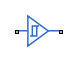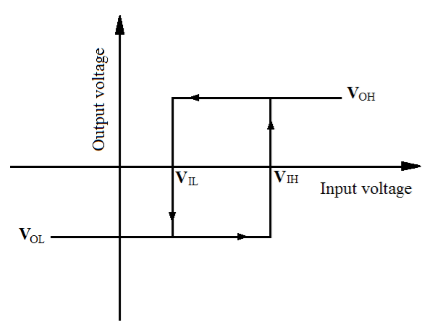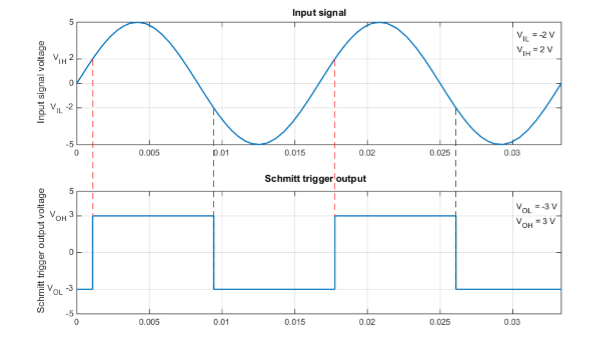# Schmitt Trigger

Behavioral model of Schmitt trigger

•Libraries:
Simscape / Electrical / Integrated Circuits / Logic

## Description

The Schmitt Trigger block implements a behavioral model of Schmitt trigger.

The block output logic level is `HIGH` when the input rises above the High level input voltage (VIH) value and does not go `LOW` until the input falls below the lower-valued Low level input voltage (VIL) value. This logic implements a hysteresis characteristic between input and output.In the graphic, VOH and VOL correspond to the High level output voltage and Low level output voltage values, respectively.

The next figure shows a sample output of the block with parameters VIH = 2V, VIL = -2V, VOH = 3V, and VOL = -3V.The block determines the logic levels of the gate inputs as follows:

• If the gate voltage is greater than the threshold voltage, the block interprets the input as logic 1.

• Otherwise, the block interprets the input as logic 0.

The threshold voltage is the voltage value at midpoint between the High level input voltage parameter value and the Low level input voltage parameter value.

Note

To improve simulation speed, the block does not model all the internal individual MOSFET devices that make up the gate. See Assumptions and Limitations for details.

The block models the gate as follows:

• The gate inputs have infinite resistance and finite or zero capacitance.

• The gate output offers a selection of two models: `Linear` and `Quadratic`. For more information, see Selecting the Output Model for Logic Blocks. Use the Output current-voltage relationship parameter to specify the output model.

• You can specify propagation delay for both output models. For `Linear` output, the block sets the value of the gate output capacitor such that the resistor-capacitor time constant equals the Propagation delay parameter value. For `Quadratic` output, the gate input demand is lagged to approximate the Propagation delay parameter value.

The block output voltage depends on the output model selected:

• For `Linear` model, output high is the High level output voltage parameter value, and output low is the Low level output voltage parameter value.

• For `Quadratic` model, the output voltage for High and Low states is a function of the output current, as explained in Quadratic Model Output and Parameters. For zero load current, output high is Vcc (the Supply voltage parameter value), and output low is zero volts.

## Assumptions and Limitations

The block does not model the internal individual MOSFET devices that make up the gate (except for the final MOSFET pair if you select the `Quadratic` option for the Output current-voltage relationship parameter). This limitation has the following implications:

• The block does not accurately model the gate's response to input noise and inputs that are around the logic threshold voltage.

• The block does not accurately model dynamic response.

For circuits that involve a feedback path around a set of logic gates, you might need to set a nonzero propagation delay on one or more gates.

This block is implemented using event equations. This means that you must provide an initial output state that is consistent with the block input at time zero. For example, if you set initial output state HIGH, but the initial input voltage is below the Low level input voltage, then the initial output stays HIGH, the state only correcting itself when the input voltage rises above the High level input voltage value.

## Ports

### Conserving

expand all

Electrical conserving port associated with the Schmitt Trigger input.

Electrical conserving port associated with the Schmitt Trigger output.

## Parameters

expand all

### Inputs

Voltage value below which the block interprets the input voltage as logic `LOW`.

Voltage value above which the block interprets the input voltage as logic `HIGH`.

Fixed capacitance that approximates the input capacitance for a MOSFET gate. The MOSFET capacitance depends on the applied voltage. When you drive this block with another gate, the Average input capacitance produces a rise time similar to that of the MOSFET. You can usually find this capacitance value on a manufacturer datasheet. Setting this value to zero may result in faster simulation times.

### Outputs

Select the output model:

• `Linear` - This is the default value.

• `Quadratic`

Voltage value at the output when the output logic level is `LOW`.

#### Dependencies

This parameter is visible only when you select `Linear` for the Output current-voltage relationship parameter.

Voltage value at the output when the output logic level is `HIGH`.

#### Dependencies

This parameter is visible only when you select `Linear` for the Output current-voltage relationship parameter.

Value of the series output resistor that is used to model the drop in output voltage resulting from the output current. You can derive this value from a datasheet by dividing the high-level output voltage by the maximum low-level output current.

#### Dependencies

This parameter is visible only when you select `Linear` for the Output current-voltage relationship parameter.

Supply voltage value applied to the gate in your circuit.

#### Dependencies

This parameter is visible only when you select `Quadratic` for the Output current-voltage relationship parameter.

The gate supply voltage for which mask data output resistances and currents are defined.

#### Dependencies

This parameter is visible only when you select `Quadratic` for the Output current-voltage relationship parameter.

A row vector [ R_OH1R_OH2 ] of two resistance values. The first value R_OH1 is the gradient of the output voltage-current relationship when the gate is logic HIGH and there is no output current. The second value R_OH2 is the gradient of the output voltage-current relationship when the gate is logic HIGH and the output current is I_OH.

#### Dependencies

This parameter is visible only when you select `Quadratic` for the Output current-voltage relationship parameter.

The resulting current when the gate is in the logic HIGH state, but the load forces the output voltage to zero.

#### Dependencies

This parameter is visible only when you select `Quadratic` for the Output current-voltage relationship parameter.

A row vector [ R_OL1R_OL2 ] of two resistance values. The first value R_OL1 is the gradient of the output voltage-current relationship when the gate is logic LOW and there is no output current. The second value R_OL2 is the gradient of the output voltage-current relationship when the gate is logic LOW and the output current is I_OL.

#### Dependencies

This parameter is visible only when you select `Quadratic` for the Output current-voltage relationship parameter.

The resulting current when the gate is in the logic LOW state, but the load forces the output voltage to the supply voltage Vcc.

#### Dependencies

This parameter is visible only when you select `Quadratic` for the Output current-voltage relationship parameter.

Time it takes for the output to swing from `LOW` to `HIGH` or `HIGH` to `LOW` after the input logic levels change.

The gradient of the voltage-current relationship for the protection diodes when forward biased.

#### Dependencies

This parameter is visible only when you select `Quadratic` for the Output current-voltage relationship parameter.

The voltage above which the protection diode is turned on.

#### Dependencies

This parameter is visible only when you select `Quadratic` for the Output current-voltage relationship parameter.

### Initial Conditions

Specify whether the initial output state of the block is `High` or `Low`. This parameter is used for both linear and quadratic output states, provided that the Propagation delay parameter is greater than zero and the Solver Configuration block does not have the Start simulation from steady state option selected.

## Version History

Introduced in R2015a